# Sine and Cosine Wave Flashcards

Back to “Basic Trigonometry” Page

Standardized tests such as the ACT will not get much more difficult than simply matching a graph to an equation. The equations for sine and cosine wave functions have two coefficients, A and B, and are expressed this way:

A{sin (Bθ)}

The coefficient A governs the amplitude of the graph (how tall it is). The coefficient B governs the period of the graph ( how long it takes to complete a cycle).

The equation for the graph below is expressed simply as y = sin θ or even simply y = sin x. The coefficients A and B are both 1.

The equation could be written as y = 1(sin)1(θ) or y = 1(sin)1(x). You can see that the maximum height of the graph is 1 and the minimum is -1; therefore, the amplitude is 1. You can also see that the graph completes a cycle in 2π, so its period is also 1.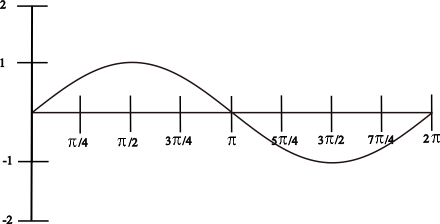You can see that the graph below begins at 1 on the y axis, which indicates a cosine wave. The amplitude is 1, and the period is 1.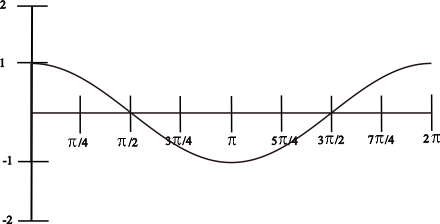When the A coefficient increases, the amplitude increases directly.

When the B coefficient increases, the period decreases inversely. So, if B=2, then the period is cut in half. If B=1/2, the period is doubled.

## One More Graph Before We Try Flashcards

See whether you can express the equation for the graph below.First, determine whether it is sine or cosine. It begins at y=0, so it is a sine wave. Next, determine the amplitude. The wave reaches up to 2 and down to -2, so the amplitude is 2.
Next, determine the period. The wave completes a cycle in 4π, so the period is 1/2. Therefore, this graph can be expressed with any of these equations:

y = 2 sin 1/2(θ)

y = 2 sin 1/2(x)

y = 2 sin x/2

## Flashcard Practice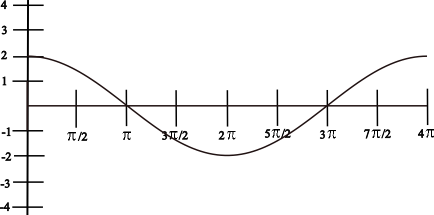• Is this wave sine or cosine?
cosine
• What is the amplitude?
2
• What is the period?
1/2
• What is the equation for this wave?
y = 2 cos x/2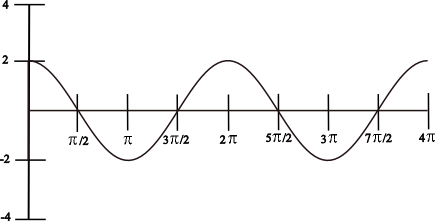• Is this wave sine or cosine?
cosine
• What is the amplitude?
2
• What is the period?
1
• What is the equation for this wave?
y = 2 cos x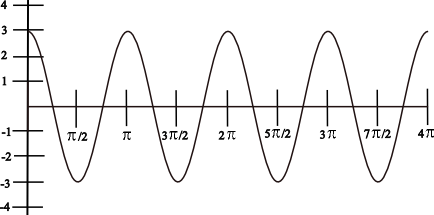• Is this wave sine or cosine?
cosine
• What is the amplitude?
3
• What is the period?
2
• What is the equation for this wave?
y = 3 cos 2x• Is this wave sine or cosine?
sine
• What is the amplitude?
3
• What is the period?
2
• What is the equation for this wave?
y = 3 sin 2x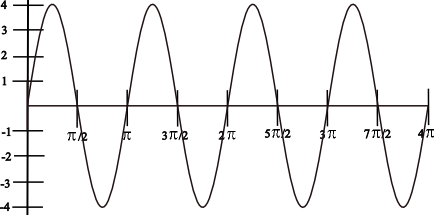• Is this wave sine or cosine?
sine
• What is the amplitude?
4
• What is the period?
2
• What is the equation for this wave?
y = 4 sin 2x

### Notice that the next three graphs are SHIFTED UPWARDS!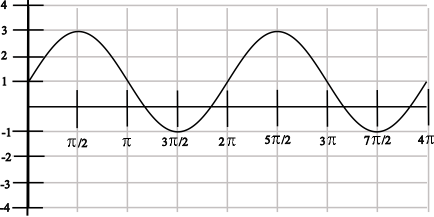• What is the period of this wave?
1
• What is the amplitude of this wave?
2
• Is this wave sine or cosine?
sine
• What is the equation for this wave?
y = 1 + 2 sin x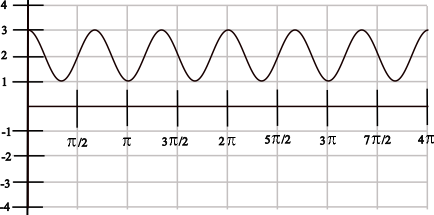Notice that this wave completes 3 cycles in 2π.

• What is the period of this wave?
3
• What is the amplitude?
1
• Is this wave sine or cosine?
cosine
• What is the equation for this wave?
y = 2 + 3 cos x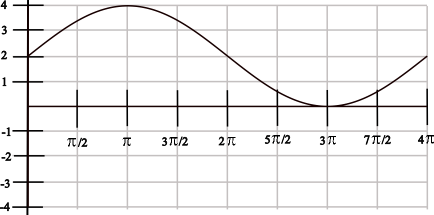Notice that this wave completes 1/2 cycle in 2π.

• What is the period of this wave?
1/2
• What is the amplitude?
2
• Is this wave sine or cosine?
sine
• What is the equation for this wave?
y = 2 + 2 sin x/2